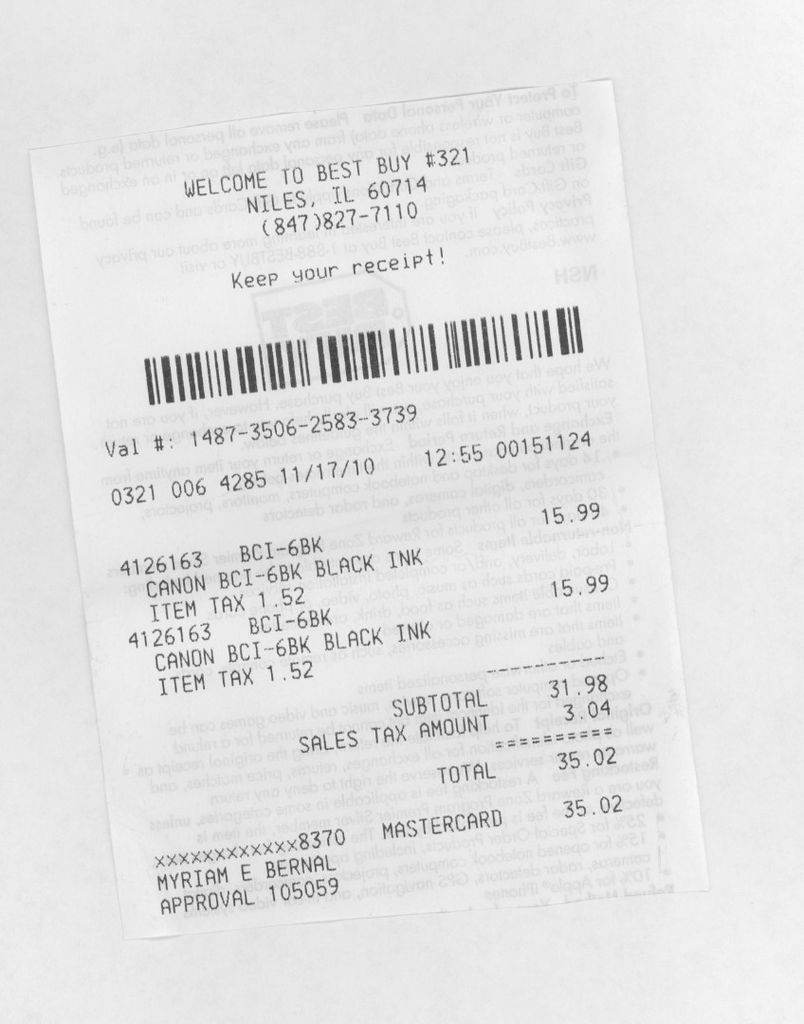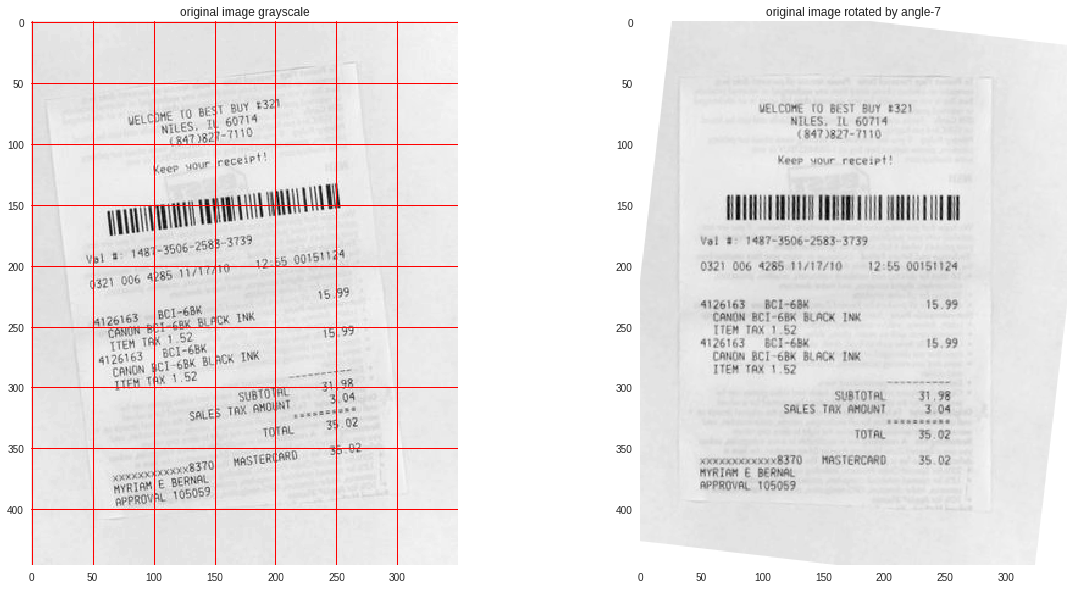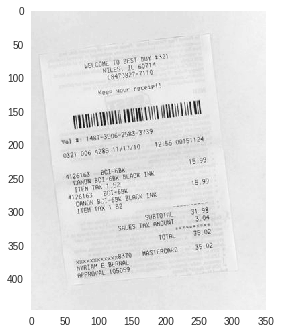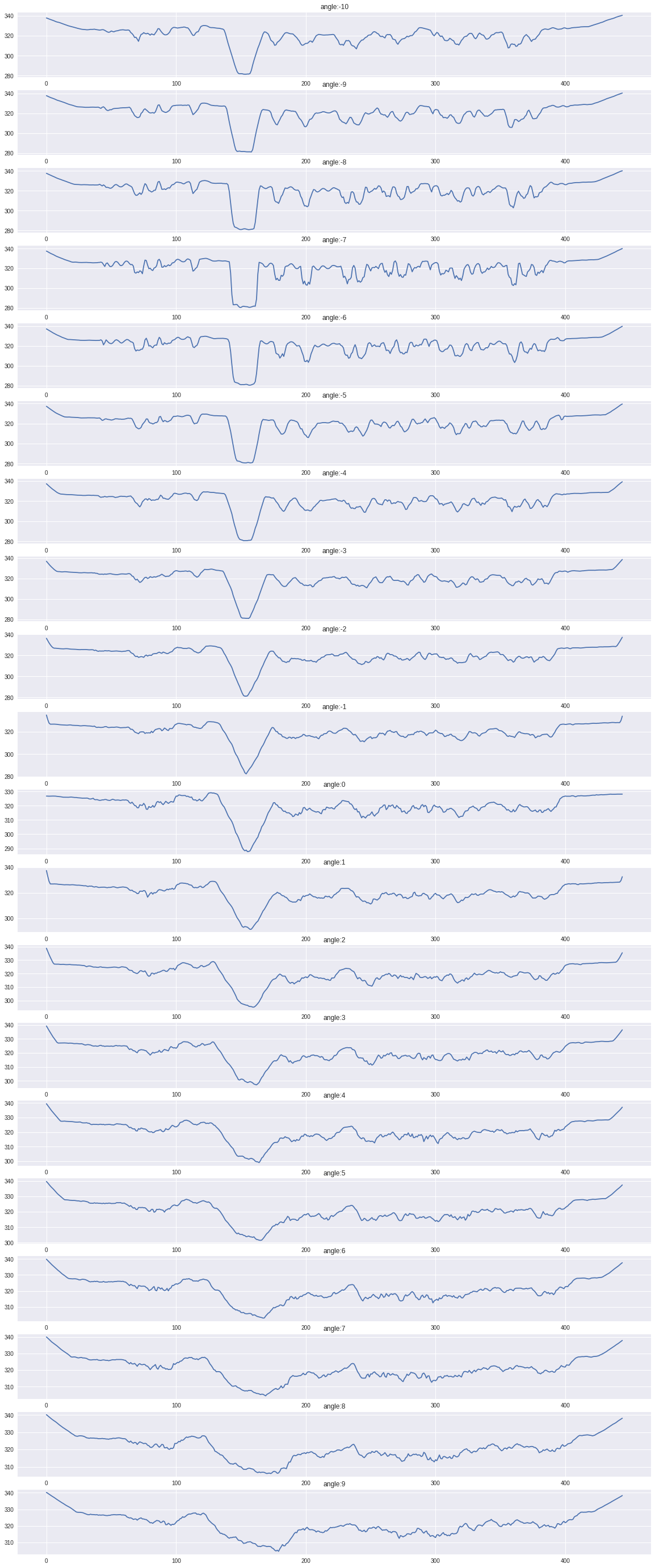# Deskewing scanned documents using horizontal projections

An important pre-processing step in any OCR tool or algorithm is to deskew the scanned document first. Take a look at the below sample scanned image, its tilted by a small angle.In this article I will explain a method to deskew these types of documents using their horizontal projections. The final outcome of deskewing will look like this:The basic steps are:

1. Convert your image to grayscale
2. Apply sobel filter
3. Find the horizontal projection array of the image at rotation angles between -10 to 10
4. Find the median of each projection array and pick the angle with the highest median.
5. Rotate the image by the identified angle.
##### Convert your image to grayscale
```from skimage.io import imread
import matplotlib.pyplot as plt
from skimage.color import rgb2gray

plt.grid()
plt.imshow(img, cmap="gray")```##### Apply Sobel filter
```from skimage.filters import sobel
from skimage.util import invert
sobel_image = invert(sobel(img))
plt.imshow(sobel_img)```I am using sobel filter just to extract the bare bones (edges) which creates this image. This process would eliminate any noise in the image.

##### Find the horizontal projection array of the image at rotation angles between -10 to 10
```def horizontal_projections(sobel_image):
sum_of_cols = []
rows,cols = sobel_image.shape
for row in range(rows-1):
sum_of_cols.append(np.sum(sobel_image[row,:]))

return sum_of_cols```As you can see in the above projections, the angle -7 degree is where we have the most number of square like signals. And since those squares depict bigger white regions, the median will be highest there. Lets see if this is true by plotting the same data on a box plot.You can clearly see how at angle -7 degree our median is the highest which takes us to our last step.

##### Find the median of each projection array and pick the angle with the highest median.
```rows,cols = sobel_image.shape
predicted_angle = 0
highest_hp = 0
for index,angle in enumerate(range(-10,10)):
hp = horizontal_projections(skimage.transform.rotate(img, angle, cval=1))
median_hp = np.median(hp)
print(median_hp)
if highest_hp < median_hp:
predicted_angle = angle
highest_hp = median_hp```
##### Rotate the image by the identified angle.
```fig, ax = plt.subplots(ncols=2, figsize=(20,10))
ax.set_title('original image grayscale')
ax.imshow(img, cmap="gray")
ax.grid(color='r', linestyle='-', markevery=1)
ax.set_title('original image rotated by angle'+str(predicted_angle))
ax.imshow(skimage.transform.rotate(img, predicted_angle, cval=1), cmap="gray")
#ax.grid(color='r', linestyle='-', markevery=1)
ax.grid(None)```You can find the entire process in this gist here.wpDiscuz